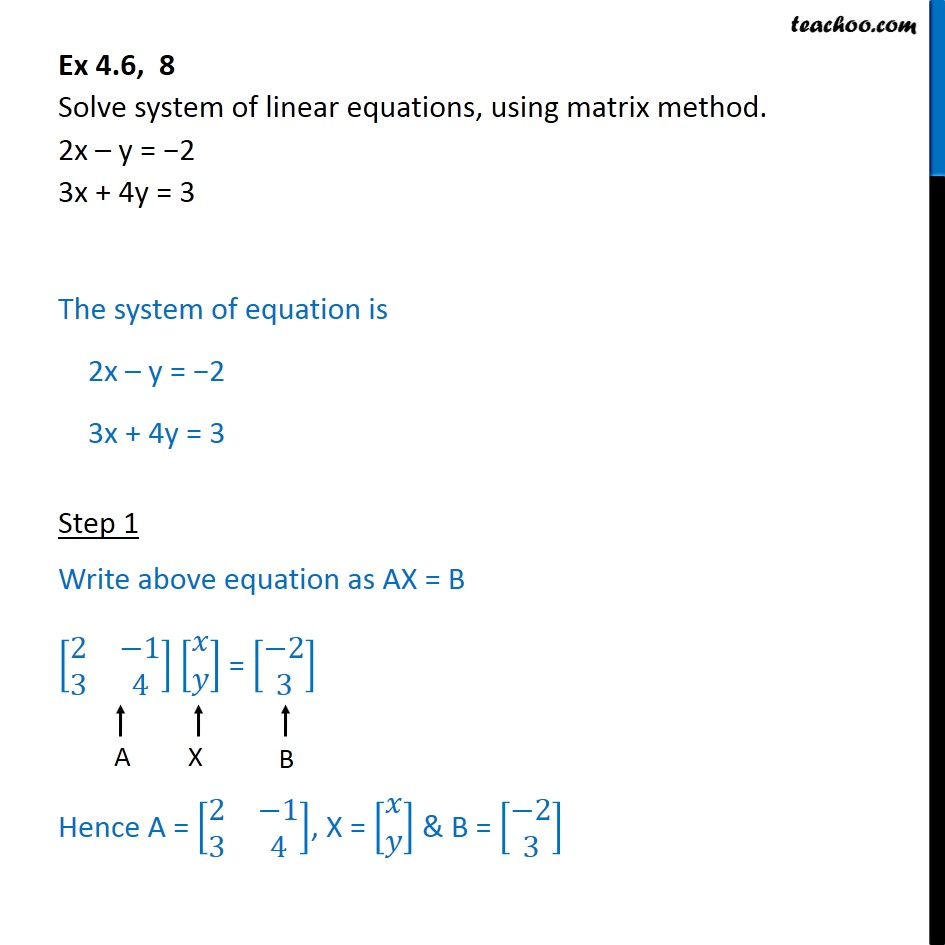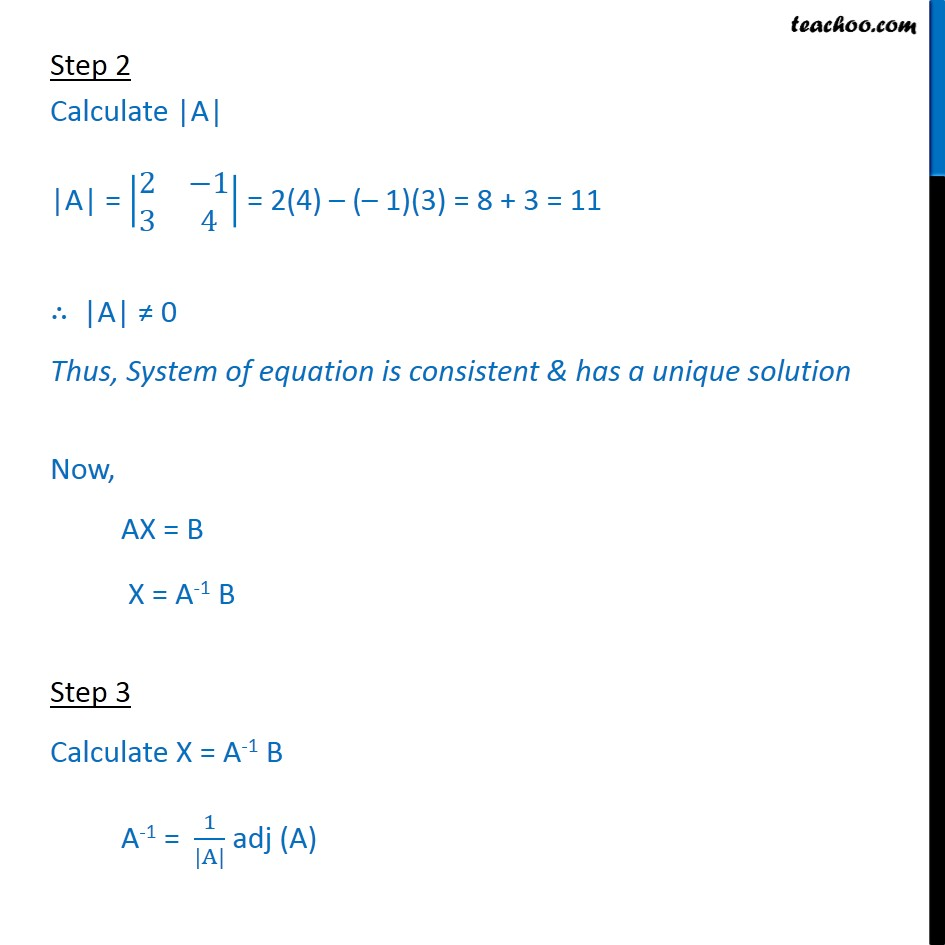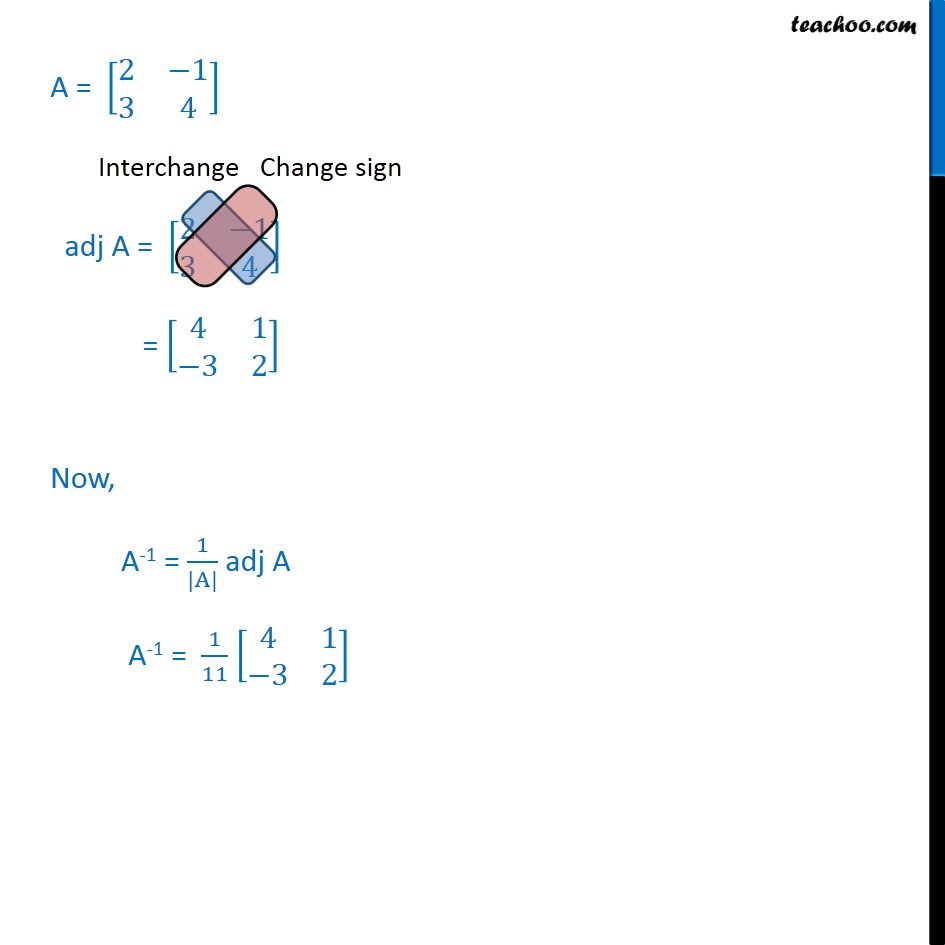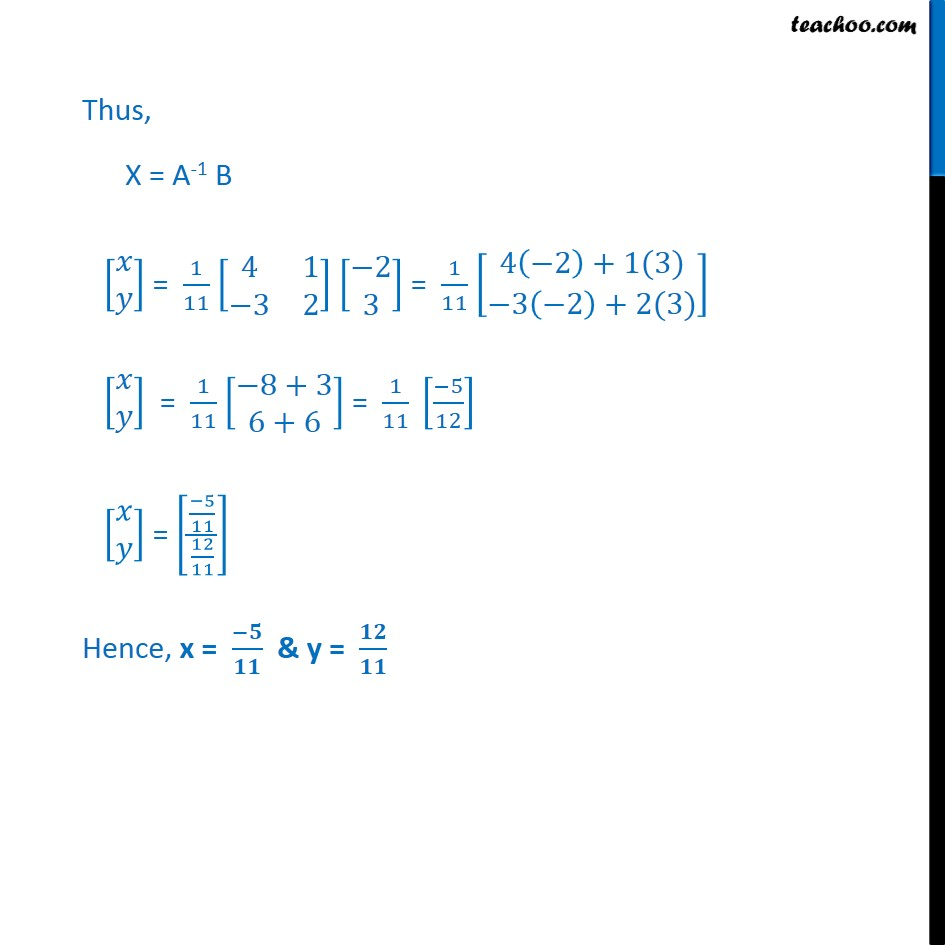Find solution of equations- Equations given

Chapter 4 Class 12 Determinants
Concept wiseLearn in your speed, with individual attention - Teachoo Maths 1-on-1 Class

### Transcript

Step 2 Calculate |A| |A| = 2 1 3 4 = 2(4) ( 1)(3) = 8 + 3 = 11 |A| 0 Thus, System of equation is consistent & has a unique solution Now, AX = B X = A-1 B Step 3 Calculate X = A-1 B A-1 = 1 |A| adj (A) A = 2 1 3 4 adj A = 2 1 3 4 = 4 1 3 2 Now, A-1 = 1 |A| adj A A-1 = 1 11 4 1 3 2 Thus, X = A-1 B = 1 11 4 1 3 2 2 3 = 1 11 4 2 +1(3) 3 2 +2(3) = 1 11 8+3 6+6 = 1 11 5 12 = 5 11 12 11 Hence, x = & y =